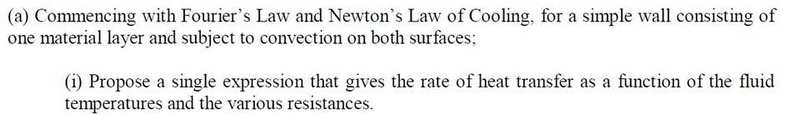# Heat transfer derivation problem

## Homework Statement## Homework Equations

Newton's law of cooling
Fourier's law

## The Attempt at a Solution

Besides writing down both the laws and perhaps drawing a diagram, I don't really know how to go about this derivation.

Any help would be really appreciated

danago
Gold Member
The diagram will probably be very helpful, but be sure to label it with all of the variables which will affect the rate of heat transfer i.e. the wall width, material conductivity, convection coefficients, far-stream temperatures etc.

If the system is in steady state, then you can conclude that the rate of heat transfer is uniform throughout the wall. You should maybe also assume that the wall is large enough in two dimensions so that "end effects" are negligible, thus reducing the problem to one in only 1-dimension.

If the far-stream temperatures are T1 and T2 and the wall surface temperature are Ts1 and Ts2, then the rates of convection on either side of the wall are (newtons law of cooling):

$$q'' = h_1 (T_1 - T_{s1} )$$

$$q'' = h_2 (T_{s2} - T_2 )$$

Since the system is in steady state and the temperature gradient through the wall will be linear, the heat flux through the wall will be (fouriers law):

$$q'' = \frac{k}{L} (T_{s1}-T_{s2})$$

You can use these three equations to derive the equation you need.